# GSEB Class 10 Maths Notes Chapter 10 Circles

This GSEB Class 10 Maths Notes Chapter 10 Circles covers all the important topics and concepts as mentioned in the chapter.

## Circles Class 10 GSEB Notes

We have studied in Class IX that a circle is a collection of all points in a plane which are at a constant distance (radius) from a fixed point (centre). We have also studied various terms related to a circle like chord, segment, sector, arc etc. Let us now examine the different situations that can arise when a circle and a line are given in a plane.

Consider a circle and a-line PQ. There can be three possibilities given in Fig. below: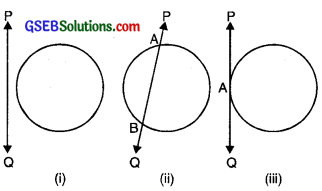In Fig. (i), the line PQ and the circle have no common point. In this case, PQ is called a non-intersecting line with respect to the circle. In Fig. (ii), there are two common points A and B that the line PQ and the circle have. In this case, we call the line PQ a secant of the circle. In Fig. (in), there is only one point A which is common to the line PQ and the circle. In this case, line is called a tangent to the circle.

We might have seen a pulley fitted over a well which is used in taking out water from the well. Look at Fig. Here the rope on both sides of the pulley, if considered as a ray, is like a tangent to the circle representing the pulley.In this chapter, we will study about the existence of the tangents to a circle and also study some of their properties.

Tangent to a Circle
In the pervious section, we have seen that a tangent to a circle is a line that intersects the circle in only one point.
The tangent to a circle is a special case of the secant, when the two end points of its corresponding chord coincide. ,
The word ‘tangent’ comes from the Latin word ‘tangere’, which means to touch and was introduced by the Danish mathematician Thomas Fineke in 1583.

The common point of the tangent and the circle is called the point of contact and the tangent is said to touch the circle at the common point.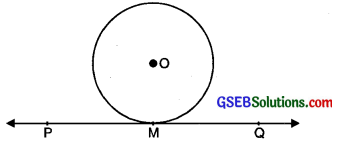Theorem 1.
The tangent at any point of a circle is perpendicular to the radius through the point of contact.
Given. A circle having O as centre. XY is tanget to the circle which meet circle at P.
To Prove. Radius is perpendicular to the tangent at a point of contact.
i. e. OP ⊥ XY.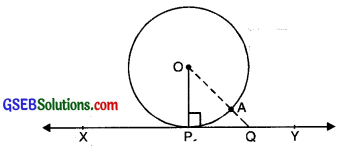Construction. Take any point on tangent XY, Q (say) which meet circle at point A on the circle. Join OQ.
Proof. In the circle. O is the centre and OP is radius of circle.
∵ Q is any point on tangent XY.
∴ Q will lie outside the circle and it meet the circle at A.
then OA < OQ
But OA = OP radii of same circle
∴ OP < OQ
Or OP is the shortest distance from point O to the tangent XY.
But, all distances from any point to any line is, perpendicular is the shortest distance.
∴ OP ⊥ XY
Hence, radius is perpendicular to the tangent.

Note:

• By theorem above, we can also conclude that at any point on a circle there can be one and only one tangent.
• The line containing the radius through the point of contact is also sometimes called the ‘normal’ to the circle at the point.

Number of Tangents from a point on a circle
According to point, we can arrange the number of tangents from a point on circle in three cases
Case 1: There is no tangent to a circle passing through a point lying inside the circle.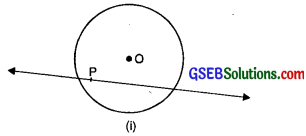Case 2: There is one and only one tangent to a circle passing through a point lying on the circle.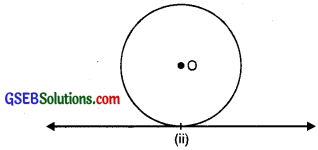Case 3: There are exactly two tangents to a circle through a point lying outside the circle.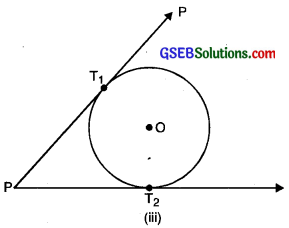In fig. (iii), T1 and T2 are the points of contact of the tangents PT1 and PT2 respectively.
The length of the segment of the tangent from the external point P and the point of contact with the circle is called the length of the tangent from the point P to the circle.Theorem 2.
The lengths of tangents drawn from an external point to a circle are equal.
Given. A circle, having as centre O and from P, any point outside the circle two tangents PQ and PR are drawn to the circle.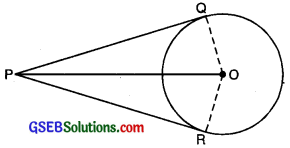To Prove: PQ = PR
Construction: Join OP
Proof: OQ is the radius and PQ is the tangent to the circle
∴ ∠PQO = 90°
[∵ a tangent to a circle is perpendicular to the radius through the point of contact]

Similarly, ∠PRO = 90°
Now, in right angle triangles PQO and PRO
∠PQO = ∠PRO = 90°
PO = PO (common side)
OQ = OR (radii of same circle)
∴ ∆PQR ≅ ∆PRO [RHS congruence]
∴ PQ = PR[CPCT]